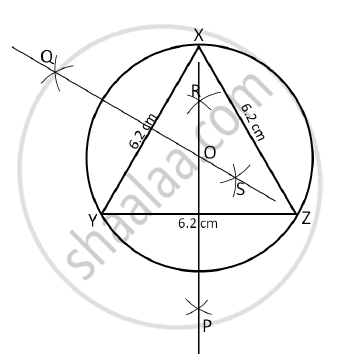# Draw an Equilateral ΔABC with Side 6.2 cm and Construct Its Circumcircle - Geometry

Draw an equilateral ΔABC with side 6.2 cm and construct its circumcircle

#### Solution

Steps of construction:
1. Construct the equilateral ΔXYZ of side equal to 6.2 cm.
2. Draw the perpendicular bisectors PR and QS of sides bar(XY) and bar(YZ) respectively.

3. Mark the point of intersection as O.
4. Draw a circle with centre O and radius OX or OY or OZ.
This is the required circumcircle.Concept: Construction of Similar Triangle
Is there an error in this question or solution?
2015-2016 (March) Set A

Share